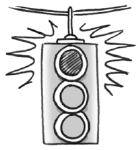### Home > A2C > Chapter 8 > Lesson 8.1.5 > Problem8-82

8-82.

Greg was working on his homework.
He completed the square to change $y = 2x^{2} − 6x + 2$ to graphing form and identify the vertex of the parabola. He did the work below and identified the vertex to be $( \frac { 3 } { 2 } , - \frac { 1 } { 4 } )$. When he got back to class and checked his answers, he discovered that his vertex was wrong, but he cannot find his mistake. Examine Greg's work and explain to him where the mistake occured. Then show him how to correct the mistake and state the vertex.

$\left. \begin{array} { l } { y = 2 x ^ { 2 } - 6 x + 2 } \\ { y = 2 ( x ^ { 2 } - 3 x ) + 2 } \\ { y = 2 ( x ^ { 2 } - 3 x + \frac { 9 } { 4 } ) + 2 - \frac { 9 } { 4 } } \\ { y = 2 ( x - \frac { 3 } { 2 } ) ^ { 2 } - \frac { 1 } { 4 } } \end{array} \right.$See problem 8-27 for help.